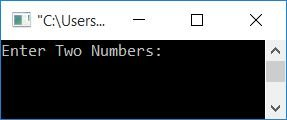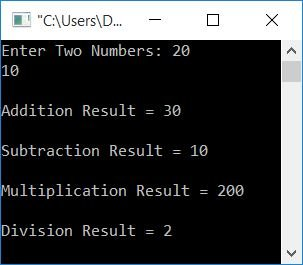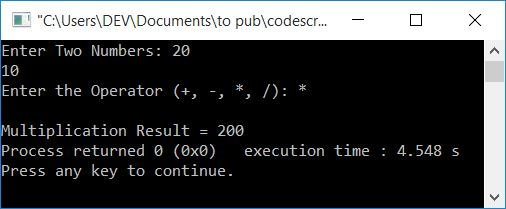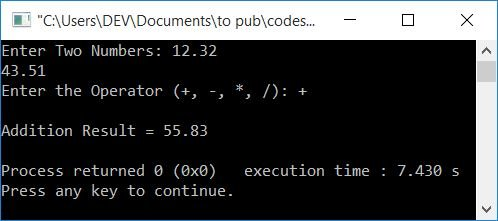# C++ program to perform addition, subtraction, multiplication, and division

Here you will learn and get code for performing addition, subtraction, multiplication, and division of any two given numbers by the user at run-time in C++ programming. Here are the approaches used to do this task:

## Add, Subtract, Multiply, and Divide in C++

To perform addition, subtraction, multiplication, and division of any two numbers in C++ programming, you have to ask the user to enter the two numbers to perform all the basic mathematical operations such as addition, subtraction, multiplication, and division. and display the result on the screen as shown in the program given below.

The subtraction and division operations go in such a way that the second number gets subtracted from the first one. Similarly, the second number divides the first one. For example, if the user enters the first and second numbers as 20 and 10, Then, the subtraction result will be 10, because 10 (the second number) gets subtracted from 20 (the first number). And the division result will be 2, because 10 divides 20.

```#include<iostream>
using namespace std;
int main()
{
int num1, num2, res;
cout<<"Enter Two Numbers: ";
cin>>num1>>num2;
res = num1+num2;
res = num1-num2;
cout<<endl<<"Subtraction Result = "<<res<<endl;
res = num1*num2;
cout<<endl<<"Multiplication Result = "<<res<<endl;
res = num1/num2;
cout<<endl<<"Division Result = "<<res<<endl;
return 0;
}```

This program was built and runs under the Code::Blocks IDE. Here is its sample run:Now supply two numbers, say 20 and 10, and press the ENTER key to see the following output:The following statement:

`cin>>num1>>num2;`

can be replaced with:

```cin>>num1;
cin>>num2;```

And the following two statements:

```res = num1+num2;

can also be replaced with only one statement:

`cout<<"\nAddition Result = "<<num1+num2<<"\n";`

In place of endl, we've used a character \n, who also begins next things on a new line.

### What if the user enters a number that contains a decimal?

To handle real numbers, change the data type from int to float. That is in place of the following statement:

`int num1, num2, res;`

Use the statement given below:

`float num1, num2, res;`

Everything else will remain the same.

## In C++, Add, Subtract, Multiply, and Divide Depending on the user's selection

Here is another program that does the same job, but instead of calculating all four operations automatically, let's ask the user what he or she wants to perform and then do the job accordingly as shown in the program given here:

```#include<iostream>
using namespace std;
int main()
{
int num1, num2;
char op;
cout<<"Enter Two Numbers: ";
cin>>num1>>num2;
cout<<"Enter the Operator (+, -, *, /): ";
cin>>op;
if(op=='+')
else if(op=='-')
cout<<endl<<"Subtraction Result = "<<num1-num2;
else if(op=='*')
cout<<endl<<"Multiplication Result = "<<num1*num2;
else if(op=='/')
cout<<endl<<"Division Result = "<<num1/num2;
else
cout<<endl<<"Wrong Operator!";
return 0;
}```

Here is its sample run with user input of two numbers as 20 and 10, and operator input as *.## In C++, Add, subtract, multiply, and divide using function

The question is: write a program in C++ that calculates the addition, subtraction, multiplication, or division of any two given numbers based on the user's choice using a user-defined function. Here is its answer:

```#include<iostream>
using namespace std;
float subFun(float, float);
float mulFun(float, float);
float divFun(float, float);
int main()
{
float num1, num2;
char op;
cout<<"Enter Two Numbers: ";
cin>>num1>>num2;
cout<<"Enter the Operator (+, -, *, /): ";
cin>>op;
if(op=='+')
else if(op=='-')
cout<<endl<<"Subtraction Result = "<<subFun(num1, num2);
else if(op=='*')
cout<<endl<<"Multiplication Result = "<<mulFun(num1, num2);
else if(op=='/')
cout<<endl<<"Division Result = "<<divFun(num1, num2);
else
cout<<endl<<"Wrong Operator!";
cout<<endl;
return 0;
}
{
return a+b;
}
float subFun(float a, float b)
{
return a-b;
}
float mulFun(float a, float b)
{
return a*b;
}
float divFun(float a, float b)
{
return a/b;
}```

Here is its sample run:#### The same program in different languages

C++ Quiz

« Previous Program Next Program »